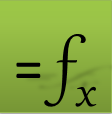## Computing Train Travel Time and Thrust

Consider a frictionless train that uses a constant propulsion force to accelerate but relies solely on the gravitational pull of the Earth and aerodynamic drag for deceleration.  Using the simplifying assumption shown in Table 1, we compute the required propulsion force and the travel time for the train between two cities 1000km  apart through a straight tunnel.

 Train mass m = 100,000 kg Distance travelled d = 1000,000 m Earth Radius Re = 6371,000 m Gravitational constant g = 10 m2/s Drag force F(v) = 0.5 v2 N Propulsion force Fp N Gravitational force Fg = mt g cos(θ) N

### Problem Formulation

Figure 1 shows the forces  acting on the train during its trip from City A to City B along the earth circular path.

The motion for the train is governed by the 2nd order equation:

$mx¨=Fp-Fdx˙+Fgθx$

where θ(x), can be approximated by the following function based on the fact that d/r << 1

$θx=tan-1Rd2-x,xd2$

The train starts from rest so the initial values are:

$x0=0$ $x˙0=0$

Upon arrival to city B, the train would have travelled distance d and come to a stop so the final conditions can be stated as:

$xtf=d$ $x˙tf=0$

The unknown variables for this problem are Fp and tf which we need to solve for.

### Solution

We treat the final conditions as constraints on the equation of motion then solve for the unknown variables Fp and tf to satisfy them. We accomplish our task following a simple three-step dynamical optimization procedure.

#### Step 1: siumlate the initial value problem using guess values for the unknowns

The first step is to convert the 2nd order equation into two first order equations as follows:

$\frac{dx}{dt}=v$ $\frac{dv}{dt}=\frac{1}{m}\left({F}_{p}-{F}_{d}\left(\stackrel{˙}{x}\right)+{F}_{g}\left(\theta \left(x\right)\right)\right)$

Next, we define the model formulas using the named variables as shown in Table 2. The green, B2:B4, and yellow, B11:B12, ranges represent the system variables (t,x,v), and the differential equations which are input arguments to the initial value problem solver function, IVSOLVE. The blue, D10:D11 range represents the design parameters Fp and tf which have been assigned initial guess values of 1000 and 2000 respectively. The other named variables and formulas in Table 2 are auxiliary variables to simplify the definition the model equations.

Variables with initial values Parameters Forces Unknowns with initial guess Equations A B C D 1 2 t m 100000 3 x 0 d 1000000 4 v 0 g 10 5 Re 6371000 6 7 Fg =m*g*COS(theta) theta =IF(xd/2,ATAN(Re/(d/2-x))+PI(),PI()/2)) 8 Fd =0.5*v^2 9 10 tf 2000 11 dx/dt =v Fp 1000 12 dv/dt =(Fp+Fd+Fg)/m

We simulate the system with the guess value for a sufficient long time of 4500 seconds by executing the array formula =IVSOLVE(B11:B12, (t,x,v), {0,4500}) in array J3:L40 shown in Table 3 and plotted in Figure 2.

Note. ExceLab 365 users pass system variables by range reference B2:B4 or as "(t,x,v)" . Google Sheets users pass by range reference or as {t,x,v}

 J K L 3 t x v 4 0 0 0 5 125 6115.463 96.66716 6 250 23618.42 180.3757 7 375 50345.74 243.5815 8 500 83614.12 285.3167 9 625 120912.9 308.8178 10 750 160244.8 318.6075 11 875 200165.6 318.7776 12 1000 239672.8 312.4788 13 1125 278105.9 301.8894 14 1250 315027.7 288.4672 15 1375 350145.5 273.1784 16 1500 383269.8 256.6382 17 1625 414271.2 239.2515 18 1750 443058.6 221.296 19 1875 469577.2 202.9315 20 2000 493781.3 184.2855 21 2125 515639.8 165.4439 22 2250 535135 146.4526 23 2375 552249.8 127.3546 24 2500 566971.2 108.1826 25 2625 579292.2 88.95507 26 2750 589207.6 69.68509 27 2875 596712.8 50.38592 28 3000 601804.6 31.06775 29 3125 604479.3 11.73845 30 3250 604737.5 -7.59494 31 3375 602579.9 -26.9254 32 3500 598007 -46.2459 33 3625 591021.5 -65.5461 34 3750 581622.5 -84.8244 35 3875 569814.4 -104.068 36 4000 555605.5 -123.255 37 4125 539004.1 -142.368 38 4250 520019.3 -161.382 39 4375 498664.3 -180.264 40 4500 474961 -198.956

#### Step 2: define constraints on the initial solution.

In Table 4, we define two constraints corresponding to the final conditions $xtf=d$ and $x˙tf=0$ based on the solution obtained in Step 1. Constraint C10 computes the difference between the interpolated value for x(tf) and the distance d, while constraint C11 computes the interpolated value for v(tf).

 C 10 =INTERPXY(J4:J40, DYNVAL(K4:K40), tf) - d 11 =INTERPXY(J4:J40, DYNVAL(L4:L40), tf)

Here we are simply computing values x(tf) and v(tf) using INTERPXY based on the result in Table 3. Note that we use DYNVAL to select the displacement and velocity columns from the solution array because we want to use their dynamic values during the optimization. The time column values are static, therefore, we can select its values directly.

Please note. DYNVAL is a new function available in ExceLab 7.0 and later versions only. It replaces deprecated Criterion Functions used in earlier versions.

#### Step 3: solve for the unknowns

Using the solver NLSOLVE, we solve for the unknowns tf and Fp by evaluating the array formula =NLSOLVE(C10:C11, (tf, Fp)) in array A10:B14. The computed results are shown in Table 5. NLSOLVE finds the best values for the variables which drive the constraint formulas values simultaneously to zero.

Note. ExceLab 365 users pass tf and Fp variables by range reference D10:D11. Google Sheets users pass by range reference or as {tf,Fp}

 A B 10 tf 3863.575 11 Fp 62648.94 12 SSERROR 1E-16 13 ITRN 6 14 TIME (s) 0.459
Question or Comment? Email us:
support @ excel-works.comExceLab: Transforming Excel into a Calculus Power House

ExceLab functions and methods are protected by USA Patents 10628634, 10114812, 9892108 and 9286286.